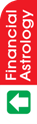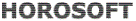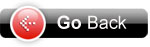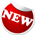Astrology Software
Indian AstrologyCalculation of Major Periods Method I : The longitude of Moon in the above example is : 6s 7° 35' 36" (6s 7° 35'). This means that Moon is in Libra (sign 7) and has travelled 7° 35' 36" (7° 35') in Libra. Find Nakshatra/star from the table given earlier. It shows that Swati Nakshatra is from 6° 40' to 20° 0'. Since our Moon is at 7° 35', it has to travel 12° 25' (20° 0' - 7° 35') more to complete Swati Nakshatra. Lord of Swati is Rahu; hence, Major Period (Mahadasha) is of Rahu at the time of birth. Rahu period will end when Moon completes Swati Nakshatra. To find out balance of Rahu period at the time of birth, proceed as under : Formula : Distance in (°) Degrees and ( ') Minutes Multiplied by Total Period of Planet Divided by 800. Hence, (12° 25') x 18 divided by 800 Where 18 is the total period of Rahu (as given above), and 800 is the total duration of one Nakshatra in minutes, i.e. we know the duration of a Nakshatra is 13° 20' = 13 x 60 + 20 = 800). For easy calculations, convert 12° 26' into minutes, i.e. 12 x 60 + 25 = 745. Hence, 745 x 18 divided by 800 = 16 305/400 years For converting 305/400 years into months, multiply by 12, = 305/400 x 12 = 9 15/100 months For converting 15/100 months into days, multiply by 30, = 15/100 x 30 = 9/2 days = 4.5 or 5 days Therefore, balance of Rahu at the time of birth is 16 yrs 9 months 5 days. (For accurate calculation, we can consider 7° 35' 36" instead of only 7° 35'.) Method II Let us suppose, Nakshatra table is not handy. To find out the balance Period, proceed as under : Convert the longitudes of Moon in minutes, i.e. 6s 7° 35' 6 x 30 = 180° Add 180° and 7° = 187° 187 x 60 = 11220' Add 11220' and 35 = 11255' Now divide by 800 (duration of one Nakshatra), i.e. 11255/800 = 14 55/800 This means that 14 nakshatras have passed and in the fifteenth Nakshatra, 55' have also passed. Fifteenth Nakshatra is Swati. We know that duration of one Nakshatra is 13° 20'. Hence, the balance of Swati Nakshatra is 13° 20' - 55' = 12° 25'. For the rest, follow the Method I. Method III : You already have the Annual Ephemeris for 1993, which will be used for calculation of Vimsottari Dasha (Major Period : Mahadasha, Period : Dasha, and Sub-period : Antar-dasha. For this purpose, Annual Ephemeris for any year can be used. In the end, different tables are given which will help us for easy calculation of the above periods. In the previous lessons, we have already calculated the Longitude of Moon. It was 6s 7° 35'. Now refer to the Table : Balance of Vimsottari Dasa by Longitude of Moon : given on page 72 of Annual Ephemeris for 1993. The Table gives the major periods in year (y), months (m) and days (d) on different Longitudes of Moon at an interval of 20'. Let us apply this to our Longitude of Moon. First of all, let us see what is the sign of our Moon. It is in 7, i.e. Libra, as it has travelled 6 signs and in the 7 sign, it has travelled 7° 35' (Already explained in the previous lesson). See that Libra (Tula) is in the fourth column (alongwith Mithuna and Kumbha). Now below this, we find Against 7° 20' Rahu 17 y 1 m 6 d ..... A This means that the major period of Rahu is on and balance is 17yrs 1 month and 6 days at 7° 20'. Now we have to find out for 7° 35', that means an addition of 15'. On page 73, there is another Table : Proportional Parts for Dasha of Planets. On the left column, number of months and days are given for each planet for 1 to 10 minutes and for 15' and 20'. We want for 15' for Rahu. See the 7th column for Rahu. Below this column, we find against 15' 4 m 2 d ..... B Subtract this (B) from the above (A), i.e. Rahu 17 y 1 m 6 d - 4 m 2 d This comes to Rahu 16 y 9 m 4 d. That means Major Period of Rahu is at the time of birth, and balance of Rahu period is 16y 9m 4d. Date of birth given above was : 10.12.1993. Balance of Rahu Major Period at time of birth : 16 y 9 m 4 d. Hence, Rahu from 10.12.1993 Add (Balance of Rahu) 4. 9. 16 This comes to : 14.9.2010 That means Rahu is from 10.12.1993 to 14.9.2010. After Rahu comes Jupiter (See above) for 16 years. Jupiter 14.9.2010 Add 16 This comes to 14.9.2026. This means Jupiter is from 14.9.2010 to 23.9.2026. Similar, calculate for other planets and write as under : Rahu 14.9.2010 Jupiter 14.9.2026 Saturn 14.9.2045 Mercury 14.9.2062 Ketu 14.9.2069 Venus 14.9.2089 Sun 14.9.2095 Moon 14.9.2105 Mars 14.9.2112 The above are the ending periods of planets.Astrowealth Astrology Software Software for Website Astrology Reports Free Services Regional Language Important Link